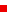## ISR Events CalendarEvent Information CCSP Seminar: Alexander Barg, "Optimal locally private estimation under $\ell_p$ loss for $1 \leq p" Thursday, February 28, 2019 5:00 p.m.-6:30 p.m. 2168 A.V. Williams Bldg. For More Information: Ajaykrishnan Nageswaran 301 405 3661 Communication, Control and Signal Processing Seminar Optimal locally private estimation under$\ell_p$loss for$1 \leq p \leq 2$Professor Alexander BargElectrical and Computer Engineering and the Institute for Systems Research AbstractWe consider the minimax estimation problem of a discrete distribution with support size$k$under locally differential privacy constraints. A privatization scheme is applied to each raw sample independently, and we need to estimate the distribution of the raw samples from the privatized samples. A positive number$\epsilon$measures the privacy level of a privatization scheme. Recently, we proposed a family of new privatization schemes and the corresponding estimator. We also proved that our scheme and estimator are order optimal in the regime$e^{\epsilon} \ll k$under both$\ell_2^2$(mean square) and$\ell_1$loss. In this talk, we sharpen this result by showing asymptotic optimality of the proposed scheme under the$\ell_p$loss for all$1 \leq p \leq 2$. More precisely, we show that for any$p \in [1,2]$and any$k$and$\epsilon$, the ratio between the worst-case$\ell_p$estimation loss of our scheme and the optimal value approaches$1$as the number of samples tends to infinity. The lower bound on the minimax risk of private estimation that we establish as a part of the proof is valid for any loss function$\ell_p, p \geq 1\$. Joint work with Min Ye (Princeton). This Event is For: Graduate • Undergraduate • Faculty • Post-DocsBrowse Events By Calendar
September 2019
SU M TU W TH F SA
1 2 3 4 5 6 7 w
8 9 10 11 12 13 14 w
15 16 17 18 19 20 21 w
22 23 24 25 26 27 28 w
29 30 w

Search Events

ISR lecture and seminar series

Submit an event to the ISR calendar Click here